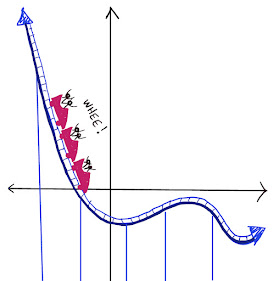## Friday, September 24, 2021

### Four fours!

Warm up:

Place operation symbols between the ones to make the equations true.  (Use the four operations and parentheses.)

1    1    1    1    =     0

1    1    1    1    =     1

1    1    1    1    =     2

1    1    1    1    =     3

1    1    1    1    =     4

Work out:

4   4   4   4  =  0

4   4   4   4  =  1

4   4   4   4  =  2

4   4   4   4  =  3

4   4   4   4  =  4

4   4   4   4  =  5

4   4   4   4  =  6

4   4   4   4  =  7

4   4   4   4  =  8

4   4   4   4  =  9

4   4   4   4  =  10

For the solution, click "Read More" below.

Solution: